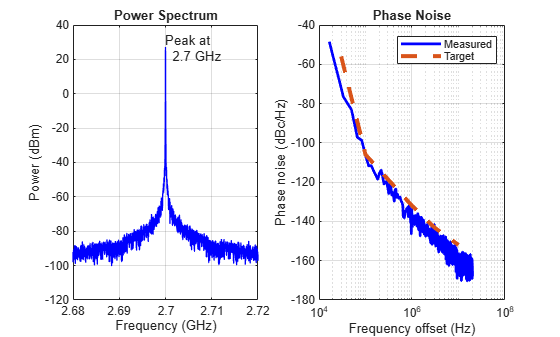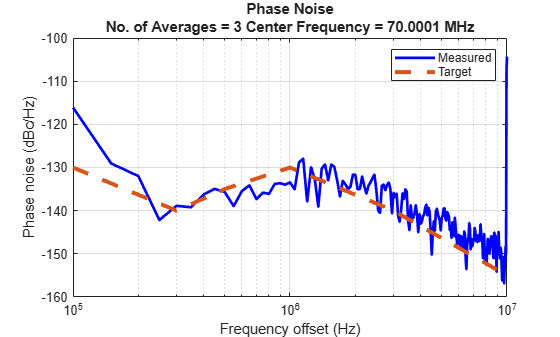# phaseNoiseMeasure

Measure and plot phase noise profile of time or frequency-domain signal

## Syntax

``PNMeasure = phaseNoiseMeasure(Xin,Yin,RBW,FrOffset,PlotOption,tag,Name,Value)``
``PNMeasure = phaseNoiseMeasure(ooooooooooooooo1`___,PNTarget,Name,Value)``
``[PNMeasure,GenFrOffset,GenPN] = phaseNoiseMeasure(___)``

## Description

````PNMeasure = phaseNoiseMeasure(Xin,Yin,RBW,FrOffset,PlotOption,tag,Name,Value)` measures the phase noise levels of either a time or frequency-domain signal at the specified frequency offset points. The function also plots phase noise profile at the specified frequency offset points when you specify the `PlotOption` argument as `'on'`. If you specify the Name-Value pair argument, enclose each argument name in quotes. Unspecified arguments take default values.```
````PNMeasure = phaseNoiseMeasure(ooooooooooooooo1`___,PNTarget,Name,Value)` in addition to the input arguments in the previous syntax compares phase noise levels at the specified frequency offsets to a target phase noise profile. Set the `PlotOption` argument to `'on'` to plot and compare the measured phase noise profile to the target profile.```
````[PNMeasure,GenFrOffset,GenPN] = phaseNoiseMeasure(___)` additionally returns the phase noise waveform data represented by the frequency offset vector and the corresponding phase noise vector.```

## Examples

collapse all

Load the power spectrum data (frequency and power vectors) of the signal obtained from spectrum analysis.

```load frequency.mat; load corresponding_power.mat;```

Set the resolution bandwidth of the spectrum analysis to 25 kHz. The frequency offset points are 30 kHz, 100 kHz, 1 MHz, 3 MHz, and 10 MHz. The target phase noise profile corresponding to these frequency offset points is:

• -56 dBc/Hz at 30 kHz

• -106 dBc/Hz at 100 kHz

• -132 dBc/Hz at 1 MHz

• -143 dBc/Hz at 3 MHz

• -152 dBc/Hz at 10 MHz

```rbw = 25e3; FrOffset = [30e3 100e3 1e6 3e6 10e6]; PNTarget = [-56 -106 -132 -143 -152];```

Use the `phaseNoiseMeasure` function to measure and plot the phase noise profile.

`PNMeasure = phaseNoiseMeasure(f1,p1,rbw,FrOffset,'on','Phase noise', PNTarget)````PNMeasure = 5×1 -70.8795 -106.2594 -136.6468 -147.3779 -157.0967 ```

Load the time domain signal represented by the time and signal value vectors.

```load time_1.mat; load signal_1.mat;```

Set the resolution bandwidth of the spectrum analysis to 75 kHz. The frequency offset points are 100 kHz, 300 kHz, 500kHz, 1 MHz, 3 MHz, and 10 MHz. The target phase noise profile corresponding to these frequency offset points is:

• -130 dBc/Hz at 100 kHz

• -140 dBc/Hz at 300 kHz

• -135 dBc/Hz at 500 kHz

• -130 dBc/Hz at 1 MHz

• -140 dBc/Hz at 3 MHz

• -155 dBc/Hz at 10 MHz

```rbw = 75e3; FrOffset = [100e3 300e3 500e3 1e6 3e6 10e6]; PNTarget = [-130 -140 -135 -130 -140 -155];```

Use the `phaseNoiseMeasure` function to measure and plot the phase noise profile.

`[PNMeasure] = phaseNoiseMeasure(t,x,rbw,FrOffset,'on','Phase noise',PNTarget,'Type','Time','Histogram','on')````PNMeasure = 6×1 -116.0967 -138.8853 -135.6704 -133.4544 -135.8667 -104.3244 ```

## Input Arguments

collapse all

Time or frequency vector, specified as a positive real vector. If you specify a time-domain signal or '`Type`' name-value pair as `'Time'`, `Xin` is a time vector in seconds. If you specify a frequency-domain signal or '`Type`' name-value pair as `'Frequency'`, `Xin` is a frequency vector in hertz.

Data Types: `double`

Signal value or power vector, specified as a real vector. If you specify a time-domain signal or '`Type`' name-value pair as `'Time'`, `Yin` is a signal value vector in volts. If you specify a frequency-domain signal or '`Type`' name-value pair as `'Frequency'`, `Yin` is a power vector in dBm.

Data Types: `double`

Resolution bandwidth used in the spectrum analysis, specified as a positive real scalar in hertz. `RBW` defines the smallest positive frequency at which the frequency components of a signal can be resolved.

Data Types: `double`

Frequency offset points at which phase noise levels are calculated, specified as a positive real vector in hertz.

Data Types: `double`

Plot the phase noise analysis results in a figure, specified as `on` or `off`. Set `PlotOption` to `'on'` to view the power spectrum and phase noise profile plots. If the Name-Value pair argument '`Type`' is specified as `'Time'`, you can only plot the phase noise profile of the time-domain signal.

Data Types: `char`

Figure identifier, specified as a string scalar or a character vector. Tag figures to keep multiple plots open as you change the simulation parameters.

Data Types: `char` | `string`

Target phase noise levels corresponding to the frequency offset points defined in `FrOffset`, specified as real vector in dBc/Hz. To compare `PNTarget` with `PNMeasure`, set `PlotOption` argument to `on` to view the phase noise comparison plot.

Data Types: `double`

### Name-Value Arguments

Specify optional comma-separated pairs of `Name,Value` arguments. `Name` is the argument name and `Value` is the corresponding value. `Name` must appear inside quotes. You can specify several name and value pair arguments in any order as `Name1,Value1,...,NameN,ValueN`.

Example: ```PNMeasure = phaseNoiseMeasure(f1,p1,rbw,FrOffset,'on','Phase noise', PNTarget,'Type','Frequency','Histogram','on')```

Type of the input signal, specified as the comma-separated consisting of `'Type'` and one of the following:

• `'Frequency'` if the input signal is power spectrum data.

• `'Time'` if the input signal is time domain data.

Plot the histogram of the half-period information for the time-domain signal, specified as the comma-separated pair consisting of `'Histogram'` and '`off`' or '`on`'. You can plot the histogram only when `'Type'` is specified as `'Time'`.

## Output Arguments

collapse all

Measured phase noise levels corresponding to frequency offset points defined in `FrOffset`, returned as a real vector in dBc/Hz. You can compare `PNMeasure` with the target phase noise levels with `PNTarget` defined in the function.

Data Types: `double`

Frequency offset points generated by the `phaseNoiseMeasure` function that are used to plot the phase noise profile, returned as a vector.

Data Types: `double`

Phase noise values generated by the `phaseNoiseMeasure` function that are used to plot the phase noise profile, returned as a vector. Each element in `GenPN` represents the phase noise at the corresponding frequency offset point represented in `GenFrOffset`.

Data Types: `double`

Introduced in R2020a

## SupportGet trial now